# Catch up on the latest AI articles# A New Explainable Artist Recommendation System Combining Knowledge Graphs And Reinforcement Learning

3 main points
✔️ Combines knowledge graph and reinforcement learning to solve the problem of recommending artists to users.
✔️ Infer the reason for the recommendation by following the agent's search path.
✔️ Better performance than the Knowledge Graph Attention Network (KGAT), which combines knowledge graphs and graph neural networks.

written by Keigo SakuraiRen TogoTakahiro OgawaMiki Haseyama
(Submitted on 30 April 2022)
Comments: Accepted by IWAIT2022
Subjects: Machine Learning (cs.LG)

The images used in this article are from the paper, the introductory slides, or were created based on them.

## first of all

With the digitization of music and the spread of music distribution services, there are more and more opportunities to listen to music by various artists. Furthermore, it is expected that digital data sales using NFT (Non-Fungible Token) will spread rapidly shortly. However, music recommendation technology in the current online market tends to be less user-oriented. For example, it is not hard to imagine that popular artists are more likely to be recommended than those with a core fan base because they contribute to sales. Therefore, more user-oriented recommendation technologies have been devised using deep learning, which has sparked the AI boom in recent years. However, deep learning has a problem that it is hard to provide a reason why an artist is recommended because deep learning generally lacks explanatory power. In this paper, we propose a new artist recommendation method that can provide reasons for the results by considering a model in which an agent explores a knowledge graph.

The novelty of this study is two points as follows.

• For proposing a new artist recommendation method that combines knowledge graphs and reinforcement learning.
• An explainability artist recommendation system by learning optimal paths on a knowledge graph.

## knowledge graph

A knowledge graph is a graph that represents information about entities and their relationships as a network structure. Note that the graph here refers to a data structure consisting of vertices and edges connecting vertices. A concrete example of a knowledge graph is the knowledge that "The capital of Japan is Tokyo. is represented by a graph in which the edge of "capital" is stretched from the vertex labeled "Japan" to the vertex labeled "Tokyo". In general, this ternary relation (triplet) is denoted as (Japan, Capital, Tokyo Metropolis). Since the knowledge graph $\mathcal{G}$ is a set of ternary relations, it can be defined by the following equation where $\mathcal{N}$ is the set of events and $\mathcal{E}$ is the set of relations.

## Search on Knowledge Graphs Using Reinforcement Learning

In our method, agents are trained to reach the artist's vertex from the user's vertex. The agent is placed at the vertex of the user to be recommended and is free to explore on the knowledge graph regardless of the orientation of the edge. We also give the following rewards to help the agent reach the appropriate artist vertex.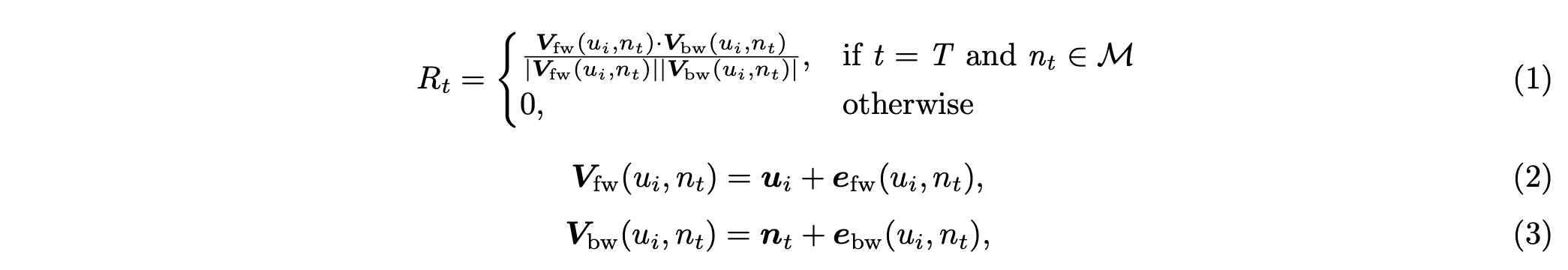where $\mathcal{M}(\in\{m_1,m_2,\dots,m_{N_m}\})$ is the set of curves, $\mathbf{e_{fw}}(u_i, n_t)$ is the sum of embedded representations of edges from vertex $u_i$ to vertex $n_t$, $\mathbf{e_{bw}}(u _i, n_t)$ denotes the sum of embedded representations of edges from vertex $n_t$ to vertex $u_i$.

The following diagram outlines the compensation calculation.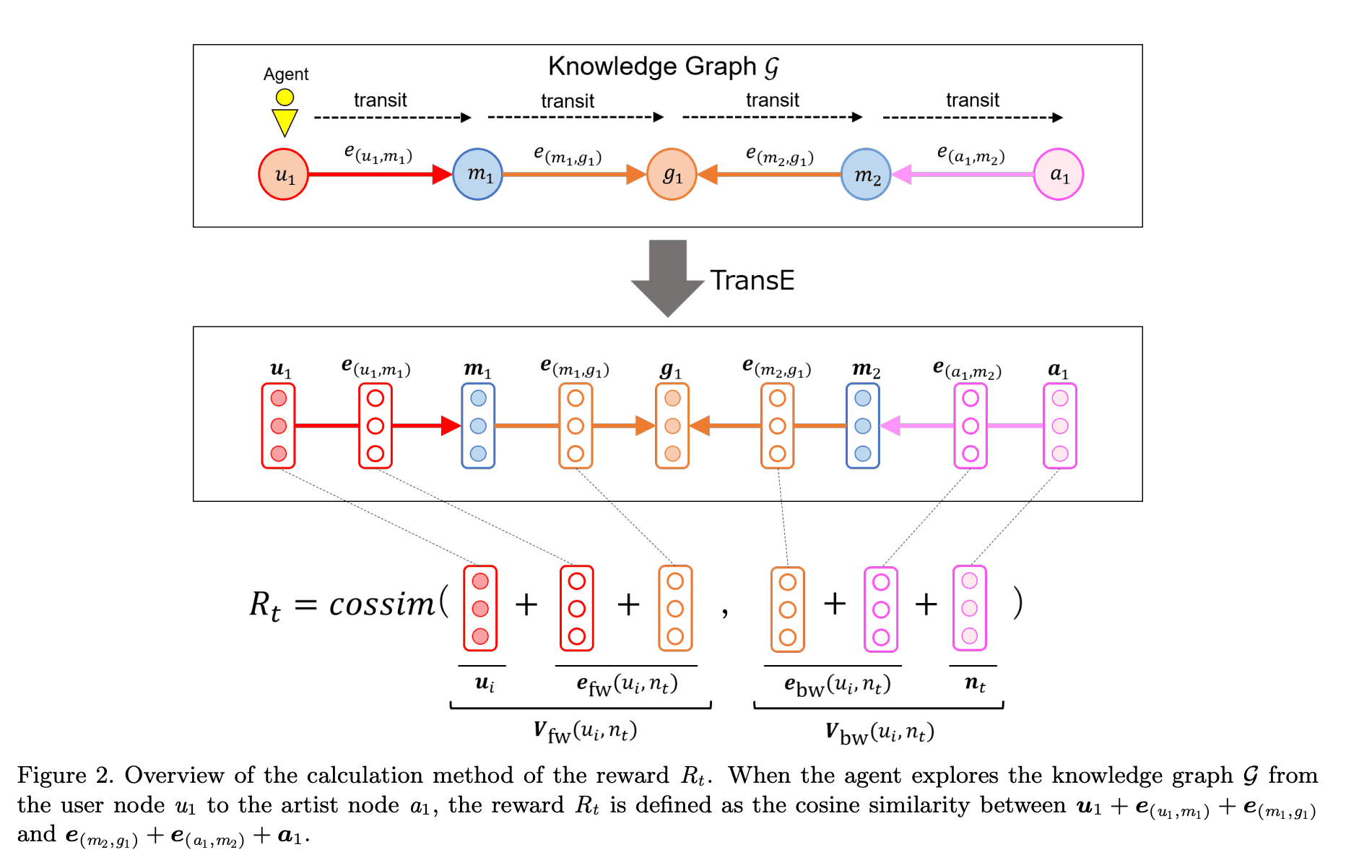In summary, this method consists of the following three steps.

1. Create knowledge graphs from data.
2. Compute the embedded representation of vertices and edges using TransE.
3. We let the agent explore the knowledge graph to find a recommendation path.

## experimental results

The experiment was evaluated using the Spotify Million Playlist Dataset. This dataset contains 57,880 songs, 1,006 users, 14,973 artists and 2,517 genres. The comparison method used was the Knowledge Graph Attention Network (KGAT), a recommendation method based on knowledge graphs and graph neural networks. The results are shown in the table below and it outperforms KGAT.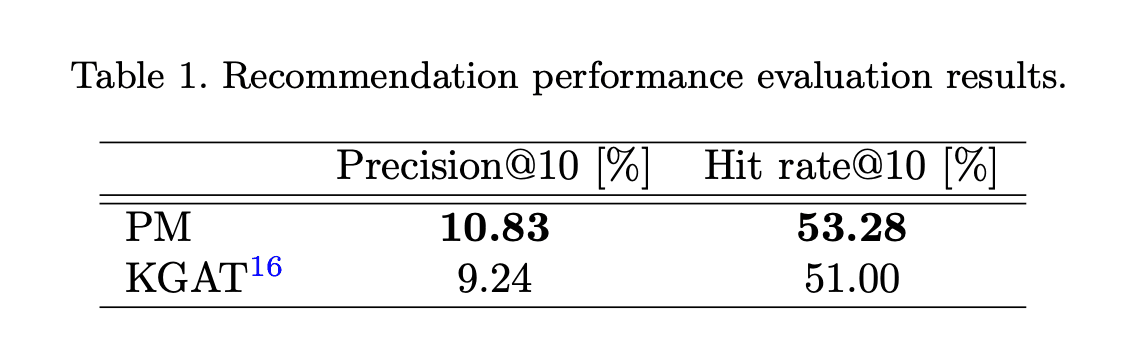The evaluation index is calculated as follows.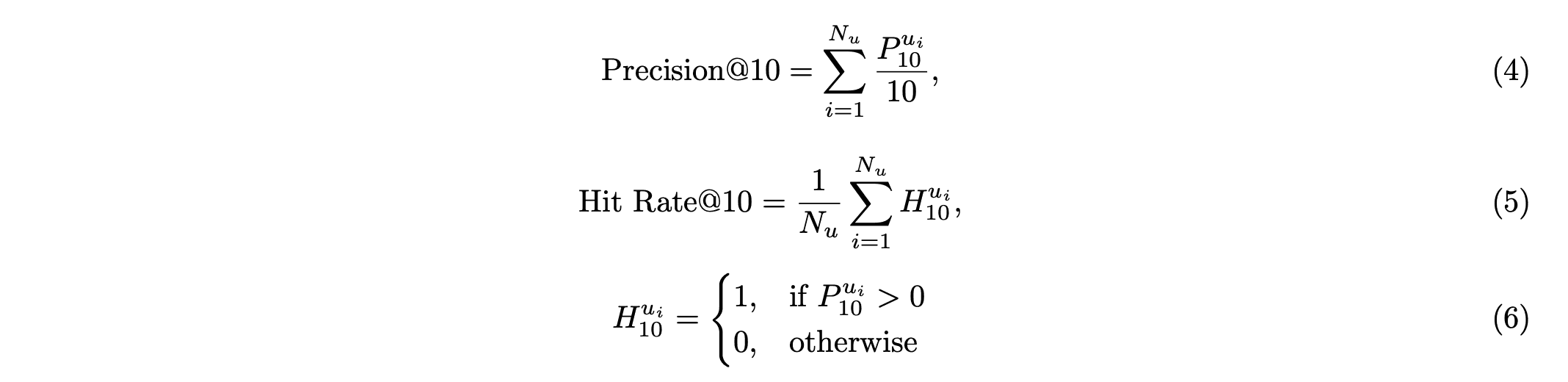where $P_{10}^{u_i}$ is the number of the top 10 artists who composed the song heard by user $u_i$ and recommended by user $u_i$.

### accountability

Figure 3 shows some examples of the paths that lead to a recommendation. In Examples 1 and 2, we recommend artists in the same genre as songs the user has heard before. In Examples 3 and 4, we recommend artists whose songs were previously heard by other users who have heard the same song. Thus, in this method, the reason for the recommendation can be inferred to some extent by looking at the path followed by the agent.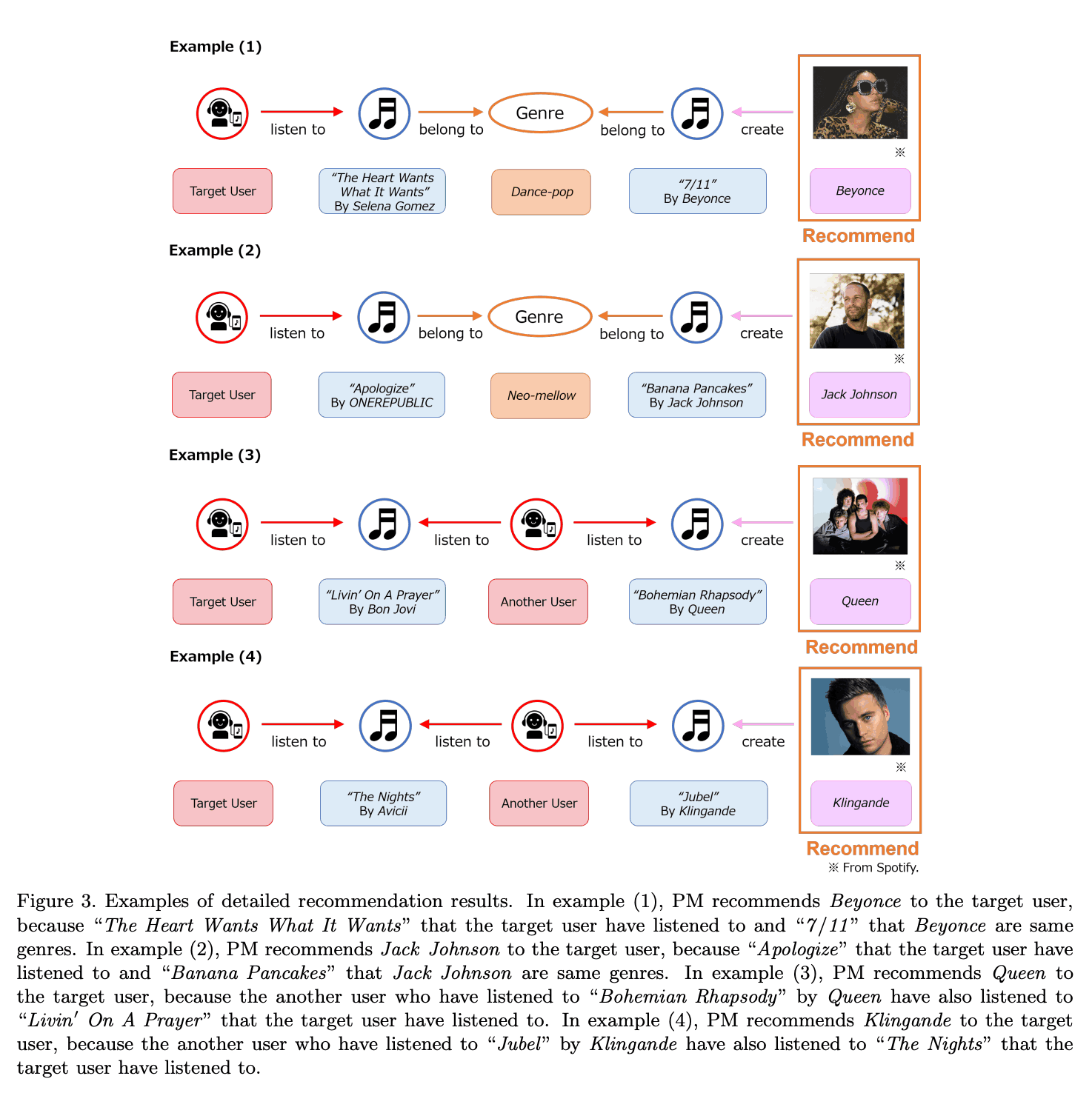## summary

We proposed a novel artist recommendation system with explainability by letting agents explore on a knowledge graph. The proposed method not only improved the explainability but also outperformed KGAT, a graph neural network-based recommendation system.

This paper shows a new direction for artist recommendation systems. However, there is room for improvement in terms of reward design and knowledge graph embedding methods, and it will be very interesting to see how the research develops in the future.

If you have any suggestions for improvement of the content of the article,
please contact the AI-SCHOLAR editorial team through the contact form.